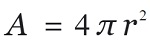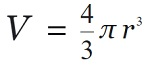Q:

# C program to calculate the surface area, volume of the Sphere

Read the radius of Sphere from the user, and then calculate the surface area and volume of the Sphere.

Surface area of the Sphere:

The formula to calculate the surface area of the Sphere is:Volume of the Sphere:

The formula to calculate the volume of the Sphere is:Program:

The source code to calculate the surface area, volume of the Sphere is given below. The given program is compiled and executed using GCC compile on UBUNTU 18.04 OS successfully.

``````// C program to calculate the surface Area and
// volume of Sphere

#include <stdio.h>
#include <math.h>

int main()
{

float volume = 0;
float area = 0;

volume = (4.0 / 3) * (3.14) * pow(radius, 3);
area = 4 * (3.14) * pow(radius, 2);

printf("Volume of Sphere 	  : %f\n", volume);
printf("Surface area of Sphere: %f\n", area);

return 0;
}``````

Output:

```RUN 1:
Volume of Sphere          : 61.569649
Surface area of Sphere: 75.391403

RUN 2:
Volume of Sphere          : 4.186666
Surface area of Sphere: 12.560000

RUN 3:
Volume of Sphere          : 5572453.500000
Surface area of Sphere: 151976.000000
```

Explanation:

Here, we read the value of radius from the user. Then we calculated the surface area and volume of the Sphere using standard formulas.